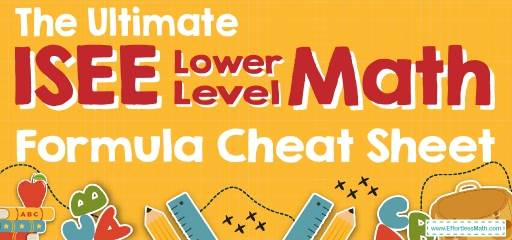# The Ultimate ISEE Lower Level Math Formula Cheat SheetIf you’re taking the ISEE Lower Level Math test in a few weeks or months, you might be anxious about how to remember ALL the different formulas and math concepts and recall them during the test.

The ISEE Lower Level Math covers a wide range of topics—from as early as elementary school to high school.

While you have probably learned many of these formulas at some point, it may have been a long time since you’ve used them. This is where most test takers have a hard time preparing for the test.

So, what formulas do you need to have memorized for the ISEE Lower Level Math before the test day?

Following is a quick formula reference sheet that lists all important ISEE Lower Level Math formulas you MUST know before you sit down for the test.

If you learn every formula in this ISEE Lower Level Math Formula Cheat Sheet, you will save yourself valuable time on the test and probably get a few extra questions correct.

Looking for a comprehensive and complete list of all ISEE Lower Level Math formulas? Please have a look at ISEE Lower Level Math Formulas.

The Absolute Best Book to Ace the ISEE Lower Level Math Test

## ISEE Lower LevelMath Cheat Sheet

### Fractions

A number expressed in the form $$\frac{a}{b}$$
Adding and Subtracting with the same denominator:
$$\frac{a}{b}+\frac{c}{b}=\frac{a+c}{b}$$
$$\frac{a}{b}-\frac{c}{b}=\frac{a-c}{b}$$
Adding and Subtracting with the different denominator:
$$\frac{a}{b}+\frac{c}{d}=\frac{ad+cb}{bd}$$
$$\frac{a}{b}-\frac{c}{d}=\frac{ad-cb}{bd}$$
Multiplying and Dividing Fractions:
$$\frac{a}{b} × \frac{c}{d}=\frac{a×c}{b×d}$$
$$\frac{a}{b} ÷ \frac{c}{d}=\frac{\frac{a}{b}}{\frac{c}{d}}=\frac{ad}{bc}$$

### Decimals

Is a fraction written in a special form? For example, instead of writing  $$\frac{1}{2}$$ you can write $$0.5$$.

### Mixed Numbers

A number is composed of a whole number and a fraction. Example: $$2 \frac{2}{ 3}$$ Converting between improper fractions and mixed numbers: $$a \frac{c}{b}=a+\frac{c}{b}= \frac{ab+ c}{b}$$

### Factoring Numbers

Factor a number means breaking it up into numbers that can be multiplied together to get the original number. Example:$$12=2×2×3$$

### Integers

$$\{…,-3,-2,-1,0,1,2,3,…\}$$
Includes: zero, counting numbers, and the negative of the counting numbers

### Order of Operations

PEMDAS
(parentheses/ exponents/ multiply/ divide/ add/ subtract)

### Ratios

A ratio is a comparison of two numbers by division. Example: $$3 : 5$$, or $$\frac{3}{5}$$

### Percentages

Use the following formula to find part, whole, or percent
part $$=\frac{percent}{100}×whole$$

### Proportional Ratios

A proportion means that two ratios are equal. It can be written in two ways:
$$\frac{a}{b}=\frac{c}{d}$$ , $$a: b = c: d$$

### Percent of Change

$$\frac{New \ Value \ – \ Old \ Value}{Old Value}×100\%$$

### Expressions and Variables

A variable is a letter that represents unspecified numbers. One may use a variable in the same manner as all other numbers: Addition: $$2+a$$: $$2$$ plus a
Subtraction: $$y-3$$  : $$y$$ minus $$3$$
Division: $$\frac{4}{x}$$  : 4 divided by $$x$$
Multiplication: $$5a$$  : $$5$$ times a

### Distributive Property

$$a(b+c)=ab+ac$$

### Equations

The values of the two mathematical expressions are equal.
$$ax+b=c$$

### Parallel and Perpendicular lines:

Parallel lines have equal slopes. Perpendicular lines (i.e., those that make a $$90^°$$ angle where they intersect) have negative reciprocal slopes: $$m_{1}$$ .$$m_{2}=-1$$.
Parallel Lines (l $$\parallel$$ m)

### Equilateral:

These triangles have three equal sides, and all three angles are $$60^\circ$$.

### Isosceles:

An isosceles triangle has two equal sides. The “base” angles (the ones opposite the two sides) are equal (see the $$45^\circ$$  triangle above).

(Square if l=w)
Area=lw

### Parallelogram

(Rhombus if l=w)
Area=lh
Regular polygons are n-sided figures with all sides equal and all angles equal.
The sum of the inside angles of an n-sided regular polygon is
$$(n-2) .180^\circ$$.

### mean:

mean: $$\frac{sum \ of \ the \ data}{of \ data \ entires}$$

### mode:

value in the list that appears most often

### Average

$$\frac{sum \ of \ terms}{number \ of \ terms}$$

The Best Books to Ace the ISEE Lower Level Math Test

### What people say about "The Ultimate ISEE Lower Level Math Formula Cheat Sheet - Effortless Math: We Help Students Learn to LOVE Mathematics"?

No one replied yet.

X
30% OFF

Limited time only!

Save Over 30%

SAVE $5 It was$16.99 now it is \$11.99